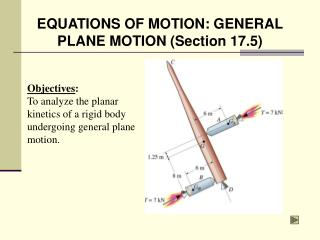DownloadDownload PresentationEQUATIONS OF MOTION: GENERAL PLANE MOTION (Section 17.5)

# EQUATIONS OF MOTION: GENERAL PLANE MOTION (Section 17.5)

Télécharger la présentation## EQUATIONS OF MOTION: GENERAL PLANE MOTION (Section 17.5)

- - - - - - - - - - - - - - - - - - - - - - - - - - - E N D - - - - - - - - - - - - - - - - - - - - - - - - - - -
##### Presentation Transcript

1. EQUATIONS OF MOTION: GENERAL PLANE MOTION (Section 17.5) Objectives: To analyze the planar kinetics of a rigid body undergoing general plane motion.

2. The forces shown on the roller’s FBD cause the accelerations shown on the kinetic diagram. = APPLICATIONS As the soil compactor accelerates forward, the front roller experiences general plane motion (both translation and rotation). What are the loads experienced by the roller shaft or bearings?

3. APPLICATIOANS (continued) During an impact, the center of gravity of this crash dummy will decelerate with the vehicle, but also experience another acceleration due to its rotation about point A. How can engineers use this information to determine the forces exerted by the seat belt on a passenger during a crash?

4. Using an x-y inertial coordinate system, the equations of motions about the center of mass, G, may be written as  Fx = m (aG)x  Fy = m (aG)y  MG = IG a P EQUATIONS OF MOTION: GENERAL PLANE MOTION When a rigid body is subjected to external forces and couple-moments, it undergoes both translational and rotational motion.

5. P EQUATIONS OF MOTION: GENERAL PLANE MOTION (continued) Sometimes, it is convenient to write the moment equation about some point P other than G. Then the equations of motion are:  Fx = m (aG)x  Fy = m (aG)y  MP =  (Mk)P Here,  (Mk )P is the sum of the moments of IGa and maG about point P.

6. FRICTIONAL ROLLING PROBLEMS When analyzing the rolling motion it may not be known if the body rolls without slipping or if it slides as it rolls. For example, consider a disk with mass m and radius r, subjected to a known force P. The equations of motion will be  Fx = m(aG)x => P - F = maG  Fy = m(aG)y => N - mg = 0  MG = IGα => F r = IG α There are 4 unknowns (F, N, α, and aG) in these three equations.

7. FRICTIONAL ROLLING PROBLEMS (continued) Hence, we need an assumption to provide another equation. The 4th equation can be obtained from the slip or non-slip condition of the disk. Case 1: Assume no slipping and use aG =a r and DO NOT use Ff = sN. After solving, you need to verify the assumption was correct by checking Ff  sN. Case 2: Assume slipping and use Ff = kN. In this case, aG  ar.